# the switch S1 is closed at time t=0

Question: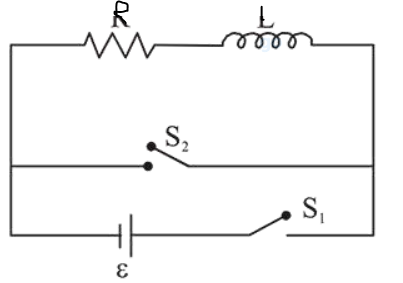the switch $S_{1}$ is closed at time $t=0$ and the switch $S_{2}$ is kept open. At some later time $\left(\mathrm{t}_{0}\right)$, the switch $\mathrm{S}_{1}$ is opened and $\mathrm{S}_{2}$ is closed. the behaviour of the current I as a function of time ' $\mathrm{t}$ ' is given by:

1. (1)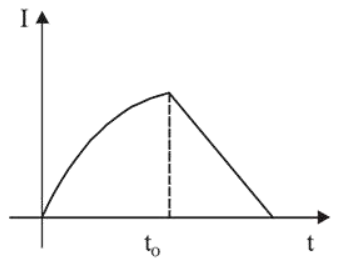2. (2)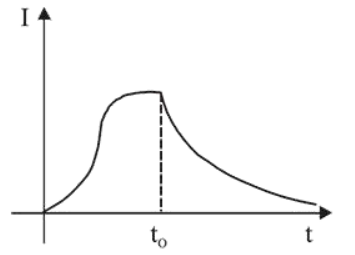3. (3)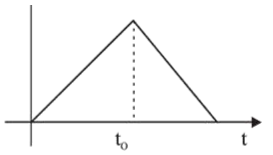4. (4)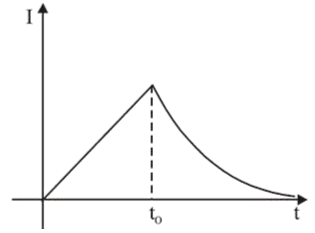Correct Option: , 2

Solution: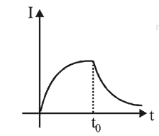The current will grow for the time $t=0$ to

$\mathrm{t}=\mathrm{t}_{0}$ and after that decay of current takes place.